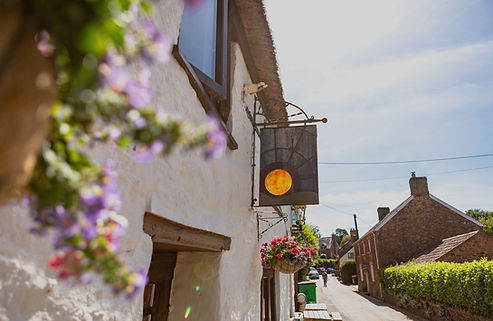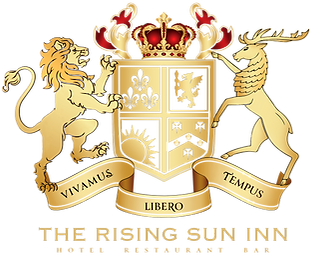# ENJOY YOUR STAY WITH US

### Cold is with the monkey's ears and toes. Cold is with the monkey's ears and toes. Cold is with the monkey's ears and toes. Cold is with the monkey's ears and toes. Cold is with the monkey's ears and toes. Cold is with the monkey's ears and toes. Cold is with the monkey's ears and toes.Cold is with the monkey's ears and toes. Cold is with the monkey's ears and toes. Cold is with the monkey's ears and toes.ROOM 1

ROOM 2

### Introduction of our non-alcoholic drinks selection. Introduction of our non-alcoholic drinks selection. Introduction of our non-alcoholic drinks selection. Introduction of our non-alcoholic drinks selection. Introduction of our non-alcoholic drinks selection.### Introduction of our chef. Introduction of our chef. Introduction of our chef. Introduction of our chef. Introduction of our chef. Introduction of our chef. Introduction of our chef. Introduction of our chef. Introduction of our chef. Introduction of our chef. Introduction of our chef. Introduction of our chef.# JOANNE BROWNJoanne

### Introduction of our wine cellar. Introduction of our wine cellar. Introduction of our wine cellar. Introduction of our wine cellar. Introduction of our wine cellar. Introduction of our wine cellar. Introduction of our wine cellar. Introduction of our wine cellar. Introduction of our wine cellar. Introduction of our wine cellar. Introduction of our wine cellar.